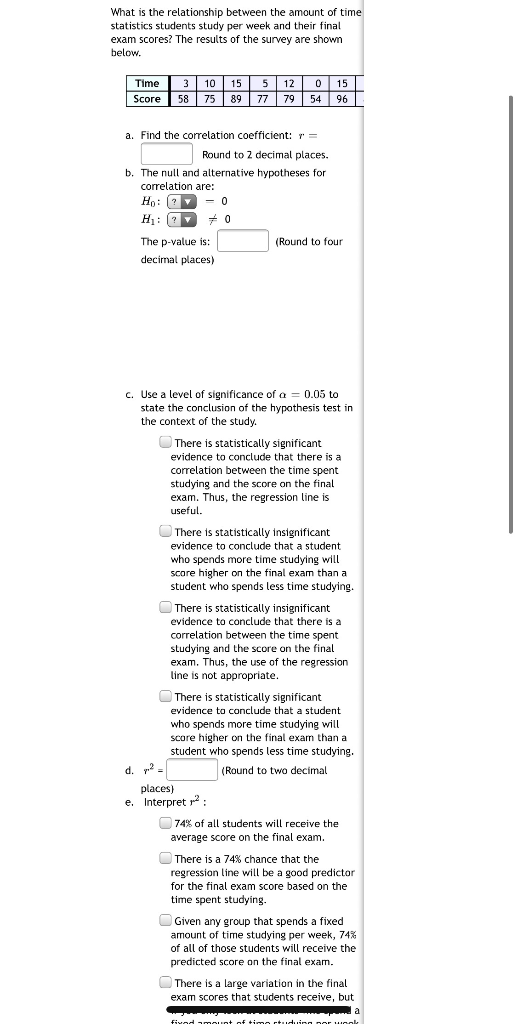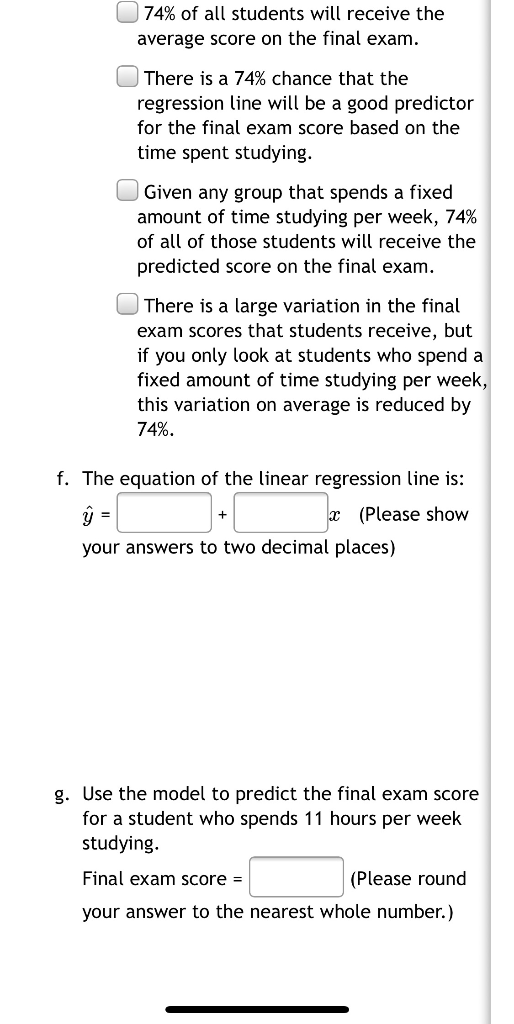# What is the relationship between the amount of time statistics students study per week and their...

###### Question:What is the relationship between the amount of time statistics students study per week and their final exam scores? The results of the survey are shown below. Time Score 3 10 15 512 015 58 75 89 89 77 79 54 96 a. Find the correlation coefficient: r = Round to 2 decimal places. b. The null and alternative hypotheses for correlation are: Ho: ? - 0 H: 70 (Round to four The p-value is: decimal places) c. Use a level of significance of a = 0.05 to state the conclusion of the hypothesis test in the context of the study. There is statistically significant evidence to conclude that there is a correlation between the time spent studying and the score on the final exam. Thus, the regression line is useful. There is statistically insignificant evidence to conclude that a student who spends more time studying will score higher on the final exam than a student who spends less time studying. There is statistically insignificant evidence to conclude that there is a correlation between the time spent studying and the score on the final exam. Thus, the use of the regression line is not appropriate. There is statistically significant evidence to conclude that a student who spends more time studying will score higher on the final exam than a student who spends less time studying. d. m? (Round to two decimal places) e. Interpret 2 74% of all students will receive the average score on the final exam. There is a 74% chance that the regression line will be a good predictor for the final exam score based on the time spent studying. Given any group that spends a fixed amount of time studying per week, 74% of all of those students will receive the predicted score on the final exam. There is a large variation in the final exam scores that students receive, but a fixed amount of time studuino por un
74% of all students will receive the average score on the final exam. There is a 74% chance that the regression line will be a good predictor for the final exam score based on the time spent studying. Given any group that spends a fixed amount of time studying per week, 74% of all of those students will receive the predicted score on the final exam. There is a large variation in the final exam scores that students receive, but if you only look at students who spend a fixed amount of time studying per week, this variation on average is reduced by 74%. f. The equation of the linear regression line is: ŷ = 2 (Please show your answers to two decimal places) g. Use the model to predict the final exam score for a student who spends 11 hours per week studying. Final exam score = (Please round your answer to the nearest whole number.)

#### Similar Solved Questions

##### 16 Consider a hypothetical X*-Y ion pair for which the equilibrium interionic spacing and bonding energy...
16 Consider a hypothetical X*-Y ion pair for which the equilibrium interionic spacing and bonding energy values are 0.38 nm and-6.13 eV,...
##### 2. (a) Explain carefully how stock returns are derived by arbitrage pricing theory (APT). (b) Briefly evaluate the empir...
2. (a) Explain carefully how stock returns are derived by arbitrage pricing theory (APT). (b) Briefly evaluate the empirical performance of APT....
##### Using Table 2 (average data of 10 brands x 10 attributes), (a) create a positioning map...
Using Table 2 (average data of 10 brands x 10 attributes), (a) create a positioning map by running SPSS, (b) put the overall mean (mean of all attributes per brand) on each brand, and (c) discuss which position must be appropriate for a new brand into the market. Table 2. Brand Positioning Mean S...
##### Exercise 6-4 Basic Segmented Income Statement [LO6-4] Royal Lawncare Company produces and sells t...
Exercise 6-4 Basic Segmented Income Statement [LO6-4] Royal Lawncare Company produces and sells two packaged products-Weedban and Greengrow. Revenue and cost information relating to the products follow: WeedbanG Selling price per unit Variable expenses per uni t Traceable fixed expenses per year ree...